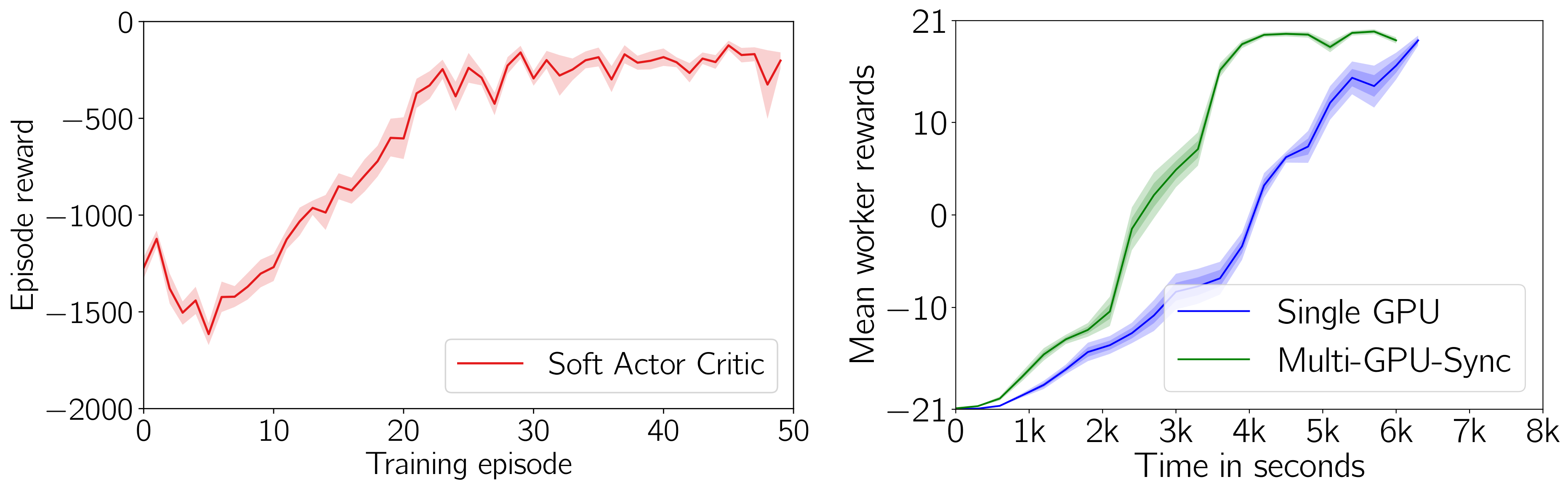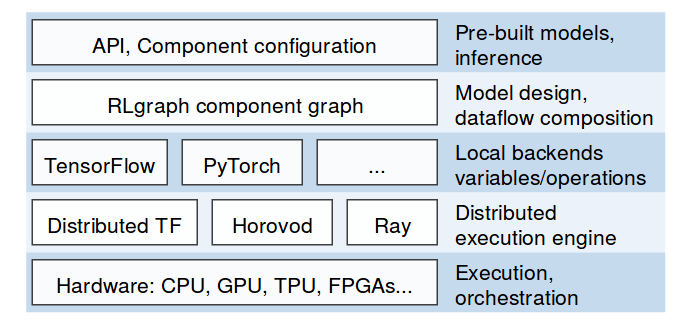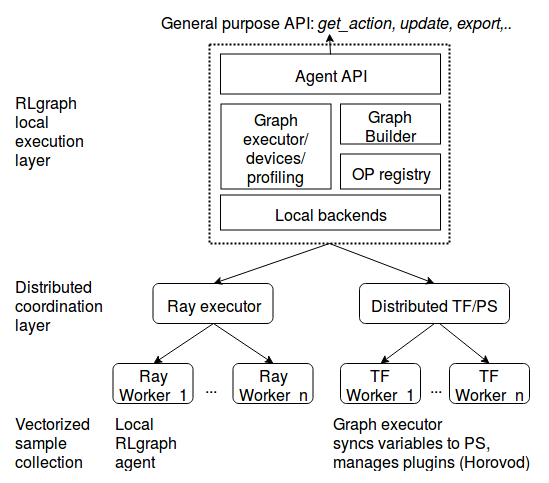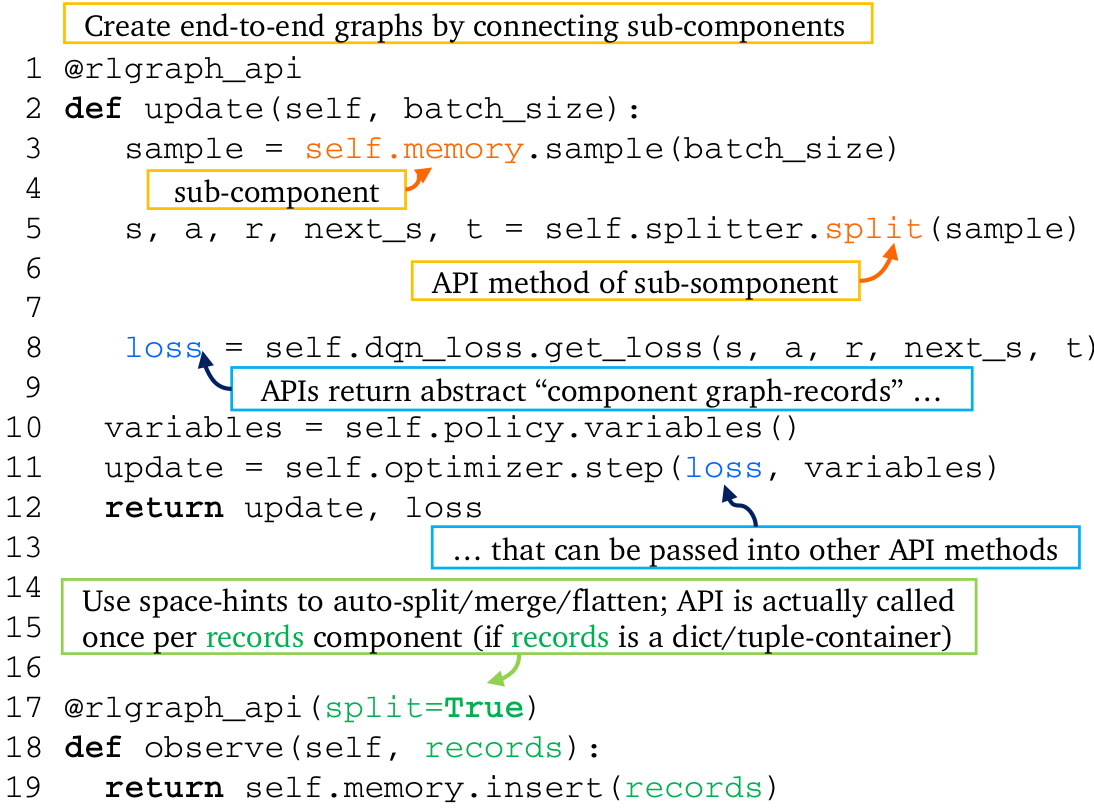# 剑桥大学等开源 RLgraph 框架，让强化学习测试可视化• 每个组件可以作为独立的图进行构建和测试，能够系统地测试复杂算法的子图，大大加快了调试和原型设计。组件可以被视为 Sonnet 风格对象，但包含 API 和设备管理、构建系统、变量创建和计算逻辑的分离。
• 后端和与空间无关的高级逻辑有助于更快地探索新设计。
• 可扩展性：RLgraph 代理可以任意执行，如：在 Ray 上使用我们的 Ray 执行包 、使用分布式 TensorFlow 来探索端到端图、或使用任意选择的分布机制。
• 维护：扩展现有框架常常意味着，不是复制大量代码就是把新的学习启发式内容不透明地堆到单个实现中。这两者都不可取。在 RLgraph 中，启发式算法是第一类公民，它们是分开构建和测试的。

## 架构## API 示例

from rlgraph.agents import agentsfrom rlgraph.spaces import * # Describe inputs via powerful space objects which manage batch and time ranks.states = IntBox(	low=0,	high=1024,	add_time_rank=True) # Nested container spaces are used throughout RLgraph# to describe state and perform shape inference.actions = Dict(	int_action=IntBox(low=0, high=5),	bool_action=BoolBox(),	float_action=FloatBox(shape=(3,)) # Create PPO agentagent = Agent.from_spec(    agent_config,    state_space=env.state_space,    action_space=env.action_space) # Use agent locally, control behaviour with flags.actions = agent.get_actions(states, use_exploration=use_exploration, apply_preprocessing=True) # Batch observe multi-environment settings.agent.observe(states, actions, rewards, terminals, batched=True , env_id="env_3") # Updates by sampling from buffer after observing.loss = agent.update() # Update from an external batch which may contain # arbitrary (non-terminal) sub-episode fragments from multiple environments,# identified via sequence indices:agent.update(batch=dict(states=states, actions=actions, rewards=rewards, terminals=terminals, sequence_indices=sequence_indices) # Single-threaded, vectorized execution. env_spec = {"type": "openai", "env_id": "CartPole-v0"}worker = SingleThreadedWorker(env_spec=env_spec, agent=agent, num_envs=8)stats = worker.execute_timesteps(100000) # Go large scale with Ray in 2 lines.ray_executor = SyncBatchExecutor(agent_config, env_spec)ray_stats = ray_executor.execute(steps=100000) # Use agent trained on Ray just as before.agent = ray_executor.local_agent

## 组件API 方法装饰器将 API 函数包装起来，以创建端到端的数据流。RLgraph 会管理每个组件的会话、变量 / 内部状态、设备、范围、占位符、嵌套、时间和批处理以及其传入传出的数据流。

record_space = Dict(    states=dict(state1=float, state2=float),    actions=dict(action1=float, action2=IntBox(10)),    # For scalar spaces, use Python primitives    reward=float,    terminals=BoolBox(),    next_states=dict(state1=float, state2=float),    add_batch_rank=True) # Memory exposes insert, sample..methodsmemory = ReplayMemory(capacity=1000)  # Input spaces contain spaces for all arguments.input_spaces = dict(records=record_space, num_records=int) # Builds the memory with variables, placeholders for these spaces.graph = ComponentTest(component=memory, input_spaces=input_spaces) # Generate a sample batch from the nested space.observation = record_space.sample(size=32) # Calls session, fetches ops, prepares inputs, executes API method.graph.test(memory.insert_records, observation)) # Get some samples providing the "num_records" int-arg.samples = graph.test((memory.insert_records, 10))

RLgraph 将张量空间和逻辑组件分开，使我们能够重用组件，而无需再次手动处理不兼容的形状。请注意，上述代码如何没有包含任何特定于框架的概念，只定义了来自一组空间的输入数据流。在 RLgraph 中，启发式算法（通常对 RL 的性能有很大的影响）不是事后才想到的，而是第一类公民，是单独或用其它组件集成测试过的。例如，策略（Policy）组件包含神经网络、动作适配器（以及相应的层次和分布）子组件，所有这些都是单独测试的。## 资源和贡献者征集

RLgraph 目前处于 alpha 阶段，正用于一些研究试点。我们欢迎贡献者和反馈。由于 RLgraph 雄心勃勃地覆盖了多个框架和后端，因此，在所有方面都有很多工作要做（我们特别欢迎更多 PyTorch 专家的加入）。